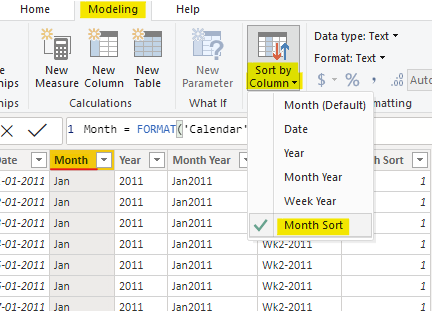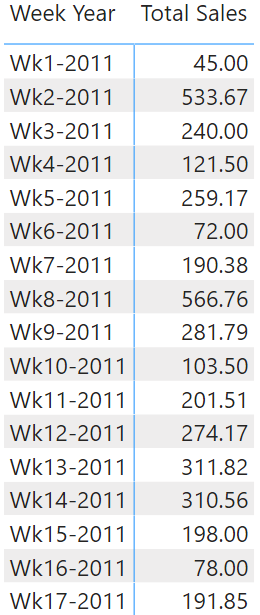Sort by Column is a great feature in Power BI that allows you to custom sort columns. You’d have mostly used this to sort months in the correct order, in this post I’ll share 4 interesting examples of Sort by Column for the Date Table.

### Glance through the Data Model real quick..

I have 2 tables: Sales and Calendar (I like calling the Date Table as Calendar)And a really simple measure for Total Sales

```Total Sales =
SUMX(
Sales,
Sales[Units] * Sales[Price]
)```

Also take a look at the columns that I have in my Calendar Table• Apart from the standard month and year columns
• I have Month Year (concat month and year) and
• Week Year (concat week number and year)

### Example 1 – Sort by Months in Power BI

Now if I place the Total Sales against the Year and Month, this is how it shows up.To fix this I create a new column in the Calendar Table with the following DAX..

`Month Sort = MONTH('Calendar'[Date])`

I then select the Month column >> Modeling Tab >> Sort by Column >> Month SortAnd now if you take a look at the visual, all months are correctly sorted.### Example 2 – Sort by Fiscal Month in Power BI

If you creating a report as per fiscal calendar you’d like the months to be sorted in the Fiscal and not Calendar Order. For instance in India the Fiscal Calendar starts with the Month of April and ends with March. I’d like that instead of the typical calendar (Jan to Dec) month order.

Think about it.. if I am able to create a column with Apr labeled as Month 1 and March as 12, I am done! I’ll create a new column (in Calendar Table) with the following DAX..

```Fiscal Month Order =
MONTH(
EDATE(
'Calendar'[Date],
-3
)
)```

Logic

• Since my first Month should be Apr, I use EDATE() to get a 3 months prior date.
• And find the month number of that.
• Select the Month Column >> Modeling Tab >> Sort by Column >> Fiscal Month Order. Done

Bingo!.. I get the months sorted from April until Mar. You can modify this logic to suit your own fiscal year.### Example 3 – Sort by Calendar Month and Year in Power BI

Notice the incorrect sorting order of Month Year• After you concat month and year, the result becomes a text (eg. Jan2011)
• And if your calendar table has dates for 2 years, you’ll have 24 distinct values in Month Year column.
• So you won’t be able to sort it by the Month number (since it only has 12 distinct values).

Logic – I am going to assign alphabets to each month starting A for Jan, B for Feb and so on… Create the following column in the Calendar Table

```Month Year Sort Order =
'Calendar'[Year] &
UNICHAR(
MONTH('Calendar'[Date])
+ 64
)```
• For the first month (Jan) the month number will be 1
• Adding 64 to 1 = 65.
• Covert 65 to an Alphabet returns A. This is the use of UNICHAR
• Again select the Month Year Column >>  Modeling Tab >> Sort by Column >> Month Year Sort Order

Done! See the results### Example 4 – Sort by Week Number and Year in Power BI

I have another column Week Year, again if I present my sales by that column, the order is collapsed. See this..I follow the same logic of assigning the Alphabets to weeks but with some twist. Consider the following constrains..

• I have 53 weeks but only 26 alphabets.
• That means after the alphabet “Z”, I’ll have to restart the order from “ZA” and so on..

Consider the following calculated column

```Week Year Sort Order =
VAR weeknum =
WEEKNUM( 'Calendar'[Date],2)
RETURN
'Calendar'[Year] &
SWITCH(
TRUE(),
weeknum <= 26,
UNICHAR(weeknum + 64),
weeknum <= 52,
"Z" & UNICHAR(weeknum + 38),
"ZZ" & UNICHAR(weeknum + 12)
)```

Logic – Assign unique alphabets to each week until 53/54 weeks. Again select Week Year >> Modeling >> Sort by Column >> Week Year Sort Order.

The results appears in order! SweetIf you have any peculiar sorting order that I haven’t discussed so far, please drop me a comment. I’d try to help 🙂